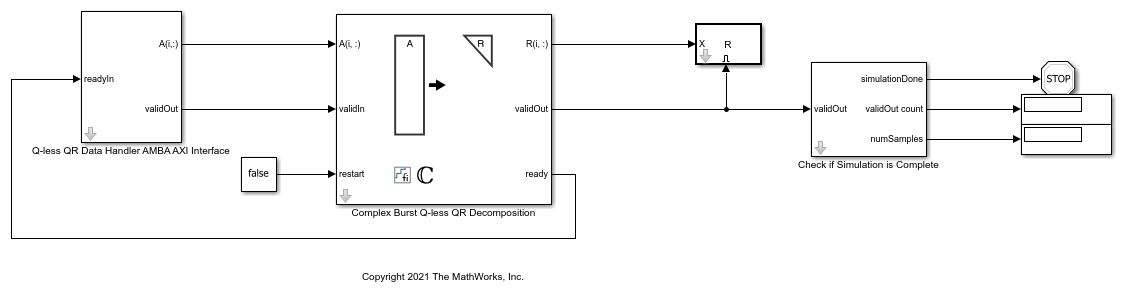# Implement Hardware-Efficient Complex Burst Q-less QR Decomposition

This example shows how to implement a hardware-efficient Q-less QR decomposition using the Complex Burst Q-less QR Decomposition block.

### Economy Size Q-less QR Decomposition

The Complex Burst Q-less QR Decomposition block performs the first step of solving the matrix equation A'AX = B which transforms A in-place to upper-triangular R, then solves the transformed system R'RX = B, where R'R = A'A.

### Define Matrix Dimensions

Specify the number of rows and columns in matrix A.

```m = 5; % Number of rows in matrix A n = 3; % Number of columns in matrix A ```

### Generate Matrix A

Use the helper function `complexUniformRandomArray` to generate a random matrix A such that the real and imaginary parts of the elements of A are between -1 and +1, and A is full rank.

```rng('default') A = fixed.example.complexUniformRandomArray(-1,1,m,n); ```

### Select Fixed-Point Data Types

Use the helper function `qlessqrFixedpointTypes` to select fixed-point data types for matrix A that guarantee no overflow will occur in the transformation of A in-place to R.

The real and imaginary parts of the elements of A are between -1 and 1, so the maximum possible absolute value of any element is sqrt(2).

```max_abs_A = sqrt(2); % Upper bound on max(abs(A(:)) precisionBits = 24; % Number of bits of precision T = fixed.qlessqrFixedpointTypes(m,max_abs_A,precisionBits); A = cast(A,'like',T.A); ```

### Open the Model

```model = 'ComplexBurstQlessQRModel'; open_system(model); ```### AMBA AXI Handshaking Process

The Data Handler subsystem in this model takes complex matrix A as input. It sends rows of A to the QR Decomposition block using the AMBA AXI handshake protocol. The `validIn` signal indicates when data is available. The `ready` signal indicates that the block can accept the data. Transfer of data occurs only when both the `validIn` and `ready` signals are high. You can set delay for the feeding in rows of A in the Data Handler to emulate the processing time of the upstream block. `validOut` signal of the Data Handler remain high when `rowDelay` is set to `0` becuase this indicates the Data Handler always has data available.

### Set Variables in the Model Workspace

Use the helper function `setModelWorkspace` to add the variables defined above to the model workspace. These variables correspond to the block parameters for the Complex Burst Q-less QR Decomposition block.

```numSamples = 1; % Number of sample matrices rowDelay = 1; % Delay of clock cycles between feeding in rows of A fixed.example.setModelWorkspace(model,'A',A,'m',m,'n',n,... 'numSamples',numSamples,'rowDelay',rowDelay); ```

### Simulate the Model

```out = sim(model); ```

### Construct the Solution from the Output Data

The Complex Burst Q-less QR Decomposition block outputs data one row at a time. When a result row is output, the block sets `validOut` to true. The rows of matrix R are output in reverse order to accommodate back-substitution, so you must reconstruct the data to interpret the results. To reconstruct the matrix R from the output data, use the helper function `qlessqrModelOutputToArray`.

```R = fixed.example.qlessqrModelOutputToArray(out.R,m,n,numSamples); ```

R is an upper-triangular matrix.

```R ```
```R = 2.1863 + 0.0000i 0.6427 - 1.0882i -0.5771 - 0.3089i 0.0000 + 0.0000i 1.8126 + 0.0000i 0.2095 + 0.0599i 0.0000 + 0.0000i 0.0000 + 0.0000i 1.7760 + 0.0000i DataTypeMode: Fixed-point: binary point scaling Signedness: Signed WordLength: 29 FractionLength: 24 ```
```isequal(R,triu(R)) ```
```ans = logical 1 ```

### Verify the Accuracy of the Output

To evaluate the accuracy of the Complex Burst Q-less QR Decomposition block, compute the relative error.

```relative_error = norm(double(R'*R - A'*A))/norm(double(A'*A)) ```
```relative_error = 8.3255e-07 ```

Suppress mlint warnings.

```%#ok<*NOPTS> ```